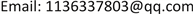1电子科技大学通信抗干扰国家级重点实验室，四川 成都

2南方电网科学研究院有限责任公司，广东 广州1. 引言

2. 系统模型2.1. 边缘计算

2.2. 基于边缘计算的电量预测模型

3. 基于梯度提升树的电量预测方法3.1. 在线学习与离线学习

3.2. 梯度提升树学习方法

L ( y , f m − 1 ( x i ) ) (1)

f m ( x i ) = f m − 1 ( x i ) − α ∂ L ( y , f m − 1 ( x i ) ) ∂ f m − 1 ( x i ) (2)

f m ( x i ) = f m − 1 ( x i ) + T ( x i ; Θ m ) (3)

T ( x i ; Θ m ) = − ∂ L ( y , f m − 1 ( x i ) ) ∂ f m − 1 ( x i ) (4)

− [ ∂ L ( y , f ( x i ) ) ∂ f ( x i ) ] f ( x ) = f m − 1 ( x ) (5)

T = { ( x 1 , y 1 ) , ( x 2 , y 2 ) , ⋯ , ( x t , y t ) } (6)

f 0 ( x ) = arg min c ∑ i = 1 t L ( y i , c ) (7)

a：对每个时刻 i = 1 , 2 , ⋯ , t 的电力数据 ( x i , y i ) ，计算当前模型负梯度：

r m i = − ∂ L ( y i , f m − 1 ( x i ) ) ∂ f m − 1 ( x i ) (8)

b：对 r m i 拟合一个CART树，得到第 m 棵树的叶节点区域 R m j ， j = 1 , 2 , ⋯ , J 。这些叶节点区域 R m j 会将训练数据集 T 分为 J 份。

c：对 j = 1 , 2 , ⋯ , J ，计算：

c m j = arg min c ∑ x i ∈ R m j L ( y i , f m − 1 ( x i ) + c ) (9)

c m j 即为能使当前模型的损失函数达到极小值的第 m 棵CART树的叶节点区域 R m j 对应的输出。

d：更新模型：

f m ( x ) = f m − 1 ( x ) + ∑ j = 1 J c m j I ( x ∈ R m j ) (10)

f M ( x ) = ∑ m = 1 M ∑ j = 1 J c m j I ( x ∈ R m j ) (11)

y ^ t + 1 = f M ( x t + 1 ) = ∑ m = 1 M ∑ j = 1 J c m j I ( x t + 1 ∈ R m j ) (12)

3.3. 基于边缘计算的电量预测流程

4. 实验验证

5. 总结